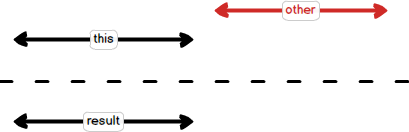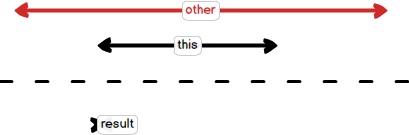# Are you sure you write OOP code?

July 20, 2020

We, PHP developers, proudly tell everybody that we know OOP and write pure OOP-code, but, honestly, more than 95% of Laravel applications code I usually see is procedural! Eloquent entities are just containers for database row data. Controllers contain methods, which just describe the procedure on how to process a web request.

Object-oriented programming is about objects which owns their data, which don't just provide their data to process by other code. Little illustration of this - question I was asked in one chat - "How can I improve this code?":

``````private function getWorkingTimeIntervals(CarbonPeriod \$businessDaysPeriod, array \$timeRanges): array
{
\$workingTimeIntervals = [];
foreach (\$timeRanges as \$time) {
\$workingTimeIntervals[] = [
'start' => Carbon::create(\$date->format('Y-m-d') . ' ' . \$time['start']),
'end' => Carbon::create(\$date->format('Y-m-d') . ' ' . \$time['end'])
];
}
}

return \$workingTimeIntervals;
}

/**
* Remove events time from working schedule
*
* @param array \$workingTimeIntervals
* @param array \$events
* @return array
*/
private function removeEventsFromWorkingTime(array \$workingTimeIntervals, array \$events): array
{
foreach (\$workingTimeIntervals as \$n => &\$interval) {
foreach (\$events as \$event) {
\$period = CarbonPeriod::create(\$interval['start'], \$interval['end']);
if (\$period->overlaps(\$event['start_date'], \$event['end_date'])) {
if (\$interval['start'] <= \$event['start_date'] && \$interval['end'] <= \$event['end_date']) {
\$interval['end'] = \$event['start_date'];
} elseif (\$interval['start'] >= \$event['start_date'] && \$interval['end'] >= \$event['end_date']) {
\$interval['start'] = \$event['end_date'];
} elseif (\$interval['start'] <= \$event['start_date'] && \$interval['end'] >= \$event['end_date']) {
\$interval['start'] = \$event['start_date'];
\$interval['end'] = \$event['end_date'];
} else {
unset(\$workingTimeIntervals[\$n]);
}
}
}
}

return \$workingTimeIntervals;
}
``````

This code works with time intervals. `removeEventsFromWorkingTime` method removes one time intervals set(events) from other(schedule) and returns the result(free schedule time). As you see, all work here is around the structure of two DateTime objects. The way how this code is working with data is very common: just some container of some data (array here) and some outside code working with this data. It's easy to duplicate some logic working with this data in the other place. Let's try to concentrate the data and logic working with this data in one object.

Each time I start a new refactoring, I try to find unit-tests for this code. Refactoring without tests might be painful. This code doesn't have them, and its structure doesn't allow to create needed tests quickly, so later, after refactoring, this functional should be checked very carefully.

``````class Interval
{
// Typed properties from PHP 7.4
public DateTimeImmutable \$start;
public DateTimeImmutable \$end;
}
``````

I'll use DateTimeImmutable as a more convenient class for working with dates. The first requirement for this class - start date should not be more than the end date. It will be much better if objects of this class couldn't be even created with invalid dates, so the best place for this check is a constructor. I want to check every requirement with unit tests. I'll explain why a bit later. First, standard constructor:

``````class Interval
{
public DateTimeImmutable \$start;
public DateTimeImmutable \$end;

public function __construct(DateTimeImmutable \$start, DateTimeImmutable \$end)
{
\$this->start = \$start;
\$this->end = \$end;
}
}
``````

Then PHPUnit tests with our requirements:

``````use App\Interval;
use PHPUnit\Framework\TestCase;

class IntervalTest extends TestCase
{
private DateTimeImmutable \$today;
private DateTimeImmutable \$yesterday;
private DateTimeImmutable \$tomorrow;

protected function setUp(): void
{
\$this->today = new DateTimeImmutable();

parent::setUp();
}

public function testValidDates()
{
\$interval = new Interval(\$this->yesterday, \$this->today);

\$this->assertEquals(\$this->yesterday, \$interval->start);
\$this->assertEquals(\$this->today, \$interval->end);
}

public function testInvalidDates()
{
\$this->expectException(\InvalidArgumentException::class);

new Interval(\$this->today, \$this->yesterday);
}
}
``````

I created some helper dates for today, yesterday, and tomorrow. The first test, `testValidDates`, just checks usual interval creation. The second one, `testInvalidDates`, checks that invalid dates provided to constructor consequence exception.
`testValidDates` will be OK, `testInvalidDates`will fail with this message:

``````Failed asserting that exception of type "InvalidArgumentException" is thrown.
``````

Let's implement this check:

``````class Interval
{
public DateTimeImmutable \$start;
public DateTimeImmutable \$end;

public function __construct(DateTimeImmutable \$start, DateTimeImmutable \$end)
{
if (\$start > \$end) {
throw new \InvalidArgumentException("Invalid date interval");
}

\$this->start = \$start;
\$this->end = \$end;
}
}
``````

Now both tests will be OK. Thanks for the modern PHP typing system, we don't have to check null or other type values. However, each time when developer writes tests, he should think about boundary conditions. The obvious boundary condition for Interval class - the same date as the start and the end of interval. Is it possible or not? That's how unit tests help to create a good design. They ask good questions before developers will ask them by themselves. Let's decide that empty interval is possible, but add a new method `isEmpty` to our class.

``````class Interval
{
public DateTimeImmutable \$start;
public DateTimeImmutable \$end;

public function __construct(DateTimeImmutable \$start, DateTimeImmutable \$end)
{
if (\$start > \$end) {
throw new \InvalidArgumentException("Invalid date interval");
}

\$this->start = \$start;
\$this->end = \$end;
}

public function isEmpty(): bool
{
return \$this->start->getTimestamp() == \$this->end->getTimestamp();
}
}

class IntervalTest extends TestCase
{
//...

public function testNonEmpty()
{
\$interval = new Interval(\$this->yesterday, \$this->today);

\$this->assertFalse(\$interval->isEmpty());
}

public function testEmpty()
{
\$interval = new Interval(\$this->today, \$this->today);

\$this->assertTrue(\$interval->isEmpty());
}
}
``````

Well, we have some basic Interval class. It can be used instead of `['start'=>,'end'=>]` arrays, but it won't make any sense. Let's add some knowledge about date intervals to our object! The base task is about creating the list of free date intervals based on work schedule and events occupying some work time. So, the first method creates an array of intervals like:

``````some day 08:00 - 12:00
some day 13:00 - 17:00
next day 08:00 - 12:00
next day 13:00 - 17:00
...
``````

The second one removes existing events from schedule:

``````Existing events:
some day 08:00 - 09:00
some day 16:00 - 17:00
next day 13:00 - 17:00

Result:
some day 09:00 - 12:00
some day 13:00 - 16:00
next day 08:00 - 12:00
...
``````

I'm going to move this logic to Interval class:

``````\$period = CarbonPeriod::create(\$interval['start'], \$interval['end']);
if (\$period->overlaps(\$event['start_date'], \$event['end_date'])) {
if (\$interval['start'] <= \$event['start_date'] && \$interval['end'] <= \$event['end_date']) {
\$interval['end'] = \$event['start_date'];
} elseif (\$interval['start'] >= \$event['start_date'] && \$interval['end'] >= \$event['end_date']) {
\$interval['start'] = \$event['end_date'];
} elseif (\$interval['start'] <= \$event['start_date'] && \$interval['end'] >= \$event['end_date']) {
\$interval['start'] = \$event['start_date'];
\$interval['end'] = \$event['end_date'];
} else {
unset(\$workingTimeIntervals[\$n]);
}
}
``````

New method `remove(Interval \$other)` will change current interval by removing other one from itself. The code will be much cleaner:

``````/**
* Remove events time from working schedule
*
* @param Interval[] \$workingTimeIntervals
* @param Interval[] \$events
* @return Interval[]
*/
private function removeEventsFromWorkingTime(\$workingTimeIntervals, \$events): array
{
foreach (\$workingTimeIntervals as \$n => \$interval) {
foreach (\$events as \$event) {
\$interval->remove(\$event);

if (\$interval->isEmpty()) {
unset(\$workingTimeIntervals[\$n]);
}
}
}

return \$workingTimeIntervals;
}
``````

Much better. Time to implement the new method. Let's start from... tests! When developers write tests, they have to think about class and method requirements and think a lot. It is useful. Let's analyze requirements by reviewing possible cases.

Interval should not be changed if `\$other` interval doesn't touch it.``````class IntervalRemoveTest extends TestCase
{
private DateTimeImmutable \$minus10Days;
private DateTimeImmutable \$today;
private DateTimeImmutable \$yesterday;
private DateTimeImmutable \$tomorrow;
private DateTimeImmutable \$plus10Days;

protected function setUp(): void
{
\$this->today = new DateTimeImmutable();
\$this->yesterday = \$this->today->sub(\DateInterval::createFromDateString("1 day"));

\$this->minus10Days = \$this->today->sub(\DateInterval::createFromDateString("10 day"));

parent::setUp();
}

public function testDifferent()
{
\$interval = new Interval(\$this->minus10Days, \$this->yesterday);

\$interval->remove(new Interval(\$this->tomorrow, \$this->plus10Days));

\$this->assertEquals(\$this->minus10Days, \$interval->start);
\$this->assertEquals(\$this->yesterday, \$interval->end);
}
}
``````

If another interval fully covers the current interval, the current one should become empty.``````class IntervalRemoveTest extends TestCase
{
public function testFullyCovered()
{
\$interval = new Interval(\$this->yesterday, \$this->tomorrow);

\$interval->remove(new Interval(\$this->minus10Days, \$this->plus10Days));

\$this->assertTrue(\$interval->isEmpty());
}

public function testFullyCoveredWithCommonStart()
{
\$interval = new Interval(\$this->yesterday, \$this->tomorrow);

\$interval->remove(new Interval(\$this->yesterday, \$this->plus10Days));

\$this->assertTrue(\$interval->isEmpty());
}

// and testFullyCoveredWithCommonEnd()
}
``````

Next, cases when another interval partially covers our interval:What if another interval placed inside?WHAAAAAT? Our interval is split to two intervals! Whole our design with `remove` method is wrong. And initial code also has a mistake here:

``````} elseif (\$interval['start'] <= \$event['start_date'] && \$interval['end'] >= \$event['end_date']) {
\$interval['start'] = \$event['start_date'];
\$interval['end'] = \$event['end_date'];
``````

I haven't even started to write the actual code but found a design problem there! This example is too trivial, and the developer could see this without tests, but it will be much harder for more complex cases. That's why unit tests are useful, they can help to write the code. Some developers say that writing code with unit tests is slower. It's true for some simple logic as the first unit tests in this article, but for complex logic, writing with unit tests might be quicker! Tests ask very good and accurate questions about your design. Developer has to create a good design.

One of the possible solutions is introducing a new class - IntervalCollection, which contains intervals and can make all operations like diff with another intervals collection:

``````class Interval
{
public DateTimeImmutable \$start;
public DateTimeImmutable \$end;

public function __construct(DateTimeImmutable \$start,
DateTimeImmutable \$end)
{
if (\$start > \$end) {
throw new \InvalidArgumentException(
"Invalid date interval");
}

\$this->start = \$start;
\$this->end = \$end;
}

public function isEmpty(): bool
{
return \$this->start === \$this->end;
}

/**
* @param Interval \$other
* @return Interval[]
*/
public function remove(Interval \$other)
{
if (\$this->start >= \$other->end
|| \$this->end <= \$other->start) return [\$this];

if (\$this->start >= \$other->start
&& \$this->end <= \$other->end) return [];

if (\$this->start < \$other->start
&& \$this->end > \$other->end) return [
new Interval(\$this->start, \$other->start),
new Interval(\$other->end, \$this->end),
];

if (\$this->start === \$other->start) {
return [new Interval(\$other->end, \$this->end)];
}

return [new Interval(\$this->start, \$other->start)];
}
}

/** @mixin Interval[] */
class IntervalCollection extends \ArrayIterator
{
public function diff(IntervalCollection \$other)
: IntervalCollection
{
/** @var Interval[] \$items */
\$items = \$this->getArrayCopy();
foreach (\$other as \$interval) {
\$newItems = [];
foreach (\$items as \$ourInterval) {
array_push(\$newItems,
...\$ourInterval->remove(\$interval));
}
\$items = \$newItems;
}

return new self(\$items);
}
}
``````

IntervalCollection class is another example of concentration of logic and data in one place, not just array with Interval classes, which will be processed by some outside functions, but a whole class with a meaningful name and covered by unit-tests.

Full source code with tests for Interval and IntervalCollection classes can be found here - https://github.com/adelf/intervals-example. The current solution's performance is super low, but it already can be used. The logic of date interval collection diff is hidden inside `IntervalCollection::diff` method and well-covered by tests. If another developer decides to optimize it, he can do it without any worries - tests will immediately catch the wrong logic! This is the second advantage of unit testing.

Organizing your code by objects which own their data helps to reduce the coupling (encapsulation and single responsibility principle are near here!). It is very important for large projects. The next step of Interval class improvement will be making it fields private.

``````class Interval
{
private DateTimeImmutable \$start;
private DateTimeImmutable \$end;

// methods
}
``````

It's possible by adding methods like `print()` which will allow seeing the values somehow, but fully restrict the work with own data from outside. But better to write another article about this.